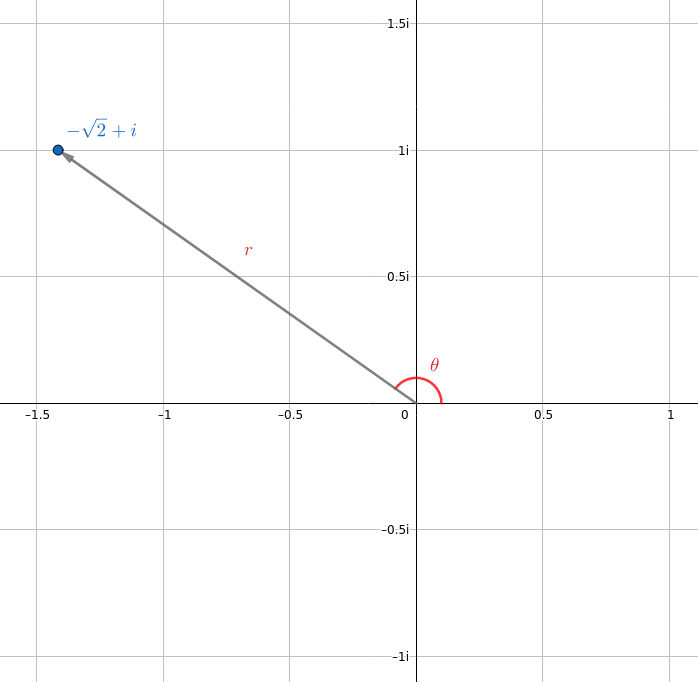Ask question

# Given w=2(cos150∘+isin150∘)w=2(cos150) and z=–sqrt{2}+i Find the polar form of z# Given w=2(cos150∘+isin150∘)w=2(cos150) and z=–sqrt{2}+i Find the polar form of z

Question
Parametric equations, polar coordinates, and vector-valued functionsasked 2021-03-02
Given $$w=2(\cos150∘+i\sin150∘)w=2(\cos150) and z=–\sqrt{2}+i$$
Find the polar form of z

## Answers (1)2021-03-03

We don't actually need ww in this case.
Remember that the polar form of a complex number is
$$z=re^{iθ}\oslash$$
where rr is the norm of z and θ angle it makes with the xx axis.Using a little trigonometry (form a right triangle using the negative xx axis, the arrow shown and a straight line segment between them), we know that
$$r = \sqrt{√2^{2}+1^{2}}=\sqrt{3}$$
and that
$$\tan(π−\oslash) = \frac{1}{\sqrt{2}}$$
$$π−\oslash=\arctan \frac{1}{\sqrt{2}}$$
$$\oslash=\pi−\arctan \frac{1}{\sqrt{2}}$$
Hence the polar form of z is
$$\boxed{z = \sqrt{3}e^{[\pi - \arctan(1/,\sqrt{2})]i}}$$
which is approximately
$$z=1.73e^{2.53i}$$

### Relevant Questionsasked 2020-11-08
Given $$\displaystyle{w}={2}{\left({\cos{{150}}}∘+{i}{\sin{{150}}}∘\right)}{w}={2}{\left({\cos{{150}}}\right)}{\quad\text{and}\quad}{z}=–\sqrt{{{2}}}+{i}$$
Find the polar form of zasked 2021-03-05
1. A curve is given by the following parametric equations. x = 20 cost, y = 10 sint. The parametric equations are used to represent the location of a car going around the racetrack. a) What is the cartesian equation that represents the race track the car is traveling on? b) What parametric equations would we use to make the car go 3 times faster on the same track? c) What parametric equations would we use to make the car go half as fast on the same track? d) What parametric equations and restrictions on t would we use to make the car go clockwise (reverse direction) and only half-way around on an interval of [0, 2?]? e) Convert the cartesian equation you found in part “a” into a polar equation? Plug it into Desmos to check your work. You must solve for “r”, so “r = ?”asked 2021-01-08
Parametric to polar equations Find an equation of the following curve in polar coordinates and describe the curve. $$x = (1 + cos t) cos t, y = (1 + cos t) sin t, 0 \leq t \leq 2\pi$$asked 2021-01-08
Investigation Consider the helix represented by the vector-valued function $$r(t)=\ <\ 2\ \cos\ t,\ 2\ \sin\ t,\ t\ >$$ (a) Write the length of the arc son the helix as a function of t by evaluating the integral $$s=\ \int_{0}^{t}\ \sqrt{[x'(u)]^{2}\ +\ [y'(u)]^{2}\ +\ [z'(u)]^{2}\ du}$$asked 2021-02-12
Find parametric equations for the tangent line to the curve with the given parametric equations at the specified point. $$x=3\ \ln(t),\ y=4t^{\frac{1}{2}},\ z=t^{3},\ (0,\ 4,\ 1)$$asked 2021-02-06
Find parametric equations for the tangent line to the curve with the given parametric equations at the specified point. $$x=e^{-8t}\ \cos(8t),\ y=e^{-8t}\ \sin(8t),\ z=e^{-8t},\ (1,\ 0,\ 1)$$asked 2020-11-23
Find the coordinates of the point on the helix for arc lengths $$s =\ \sqrt{5}\ and\ s = 4$$. Consider the helix represented investigation by the vector-valued function $$r(t)=\ <\ 2\ \cos\ t,\ 2\ \sin\ t,\ t\ >$$.asked 2021-03-18
Write a short paragraph explaining this statement. Use the following example and your answers Does the particle travel clockwise or anticlockwise around the circle? Find parametric equations if the particles moves in the opposite direction around the circle. The position of a particle is given by the parametric equations $$x = sin t, y = cos t$$ where 1 represents time. We know that the shape of the path of the particle is a circle.asked 2020-11-17
Write a short paragraph explaining this statement. Use the following example and your answers How long does it take the particle to go once around the circle? Find parametric equations if the particle moves twice as fast around the circle. The position of a particle is given by the parametric equations $$x = sin t, y = cos t$$ where 1 represents time. We know that the shape of the path of the particle is a circle.asked 2021-03-01
Parametric equations and a value for the parameter t are given $$x = (60 cos 30^{\circ})t, y = 5 + (60 sin 30^{\circ})t - 16t2, t = 2$$. Find the coordinates of the point on the plane curve described by the parametric equations corresponding to the given value of t.
...